You are currently offline. Some features of the site may not work correctly.

# Skolem problem

In mathematics, the Skolem problem is the problem of determining whether the values of a constant-recursive sequence include the number zero. The… Expand
Wikipedia

## Papers overview

Semantic Scholar uses AI to extract papers important to this topic.
2020
2020
• ISSAC
• 2020
• Corpus ID: 219687055
The Skolem Problem asks, given a linear recurrence sequence (un), whether there exists n ∈ N such that un = 0. In this paper we… Expand
Is this relevant?
2016
2016
• ICALP
• 2016
• Corpus ID: 6886755
The Continuous Skolem Problem asks whether a real-valued function satisfying a linear differential equation has a zero in a given… Expand
•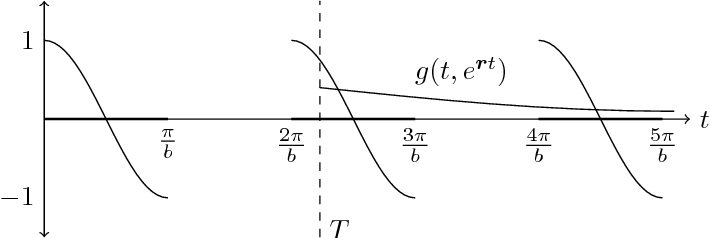Is this relevant?
2015
2015
• ArXiv
• 2015
• Corpus ID: 14220136
The Continuous Skolem Problem asks whether a real-valued function satisfying an ordinary linear differential equation has a zero… Expand
Is this relevant?
2015
2015
• Inf. Process. Lett.
• 2015
• Corpus ID: 9967553
We place the continuous-time orbit problem in P , sharpening the decidability result shown by Hainry 7. We revisit the continuous… Expand
Is this relevant?
2015
2015
• Inf. Process. Lett.
• 2015
• Corpus ID: 14525526
We consider the following decision problem: given a finite Markov chain with distinguished source and target states, and given a… Expand
•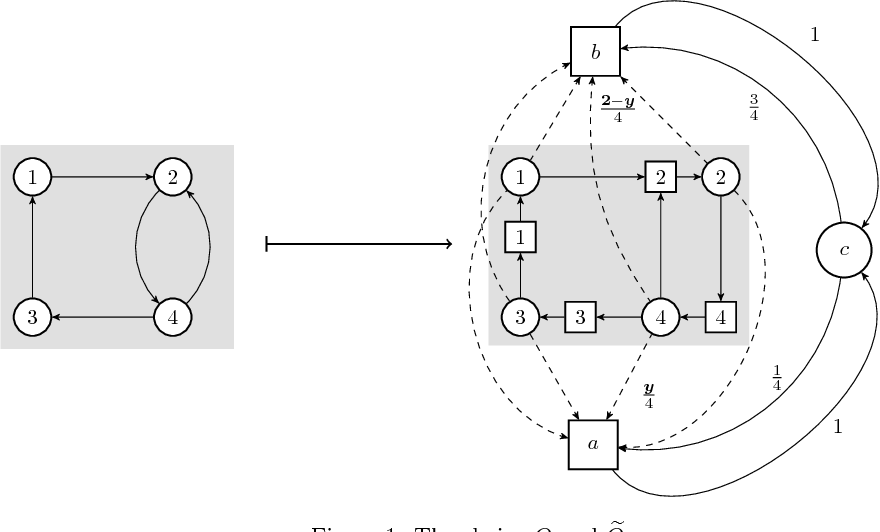Is this relevant?
2014
2014
• TOCL
• 2014
• Corpus ID: 6596047
When studying probabilistic dynamical systems, temporal logic has typically been used to analyze path properties. Recently, there… Expand
•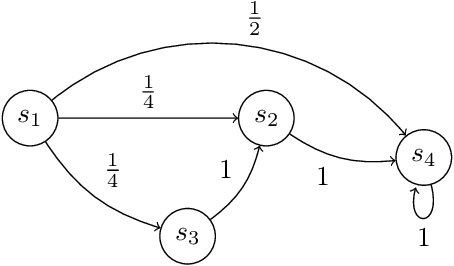•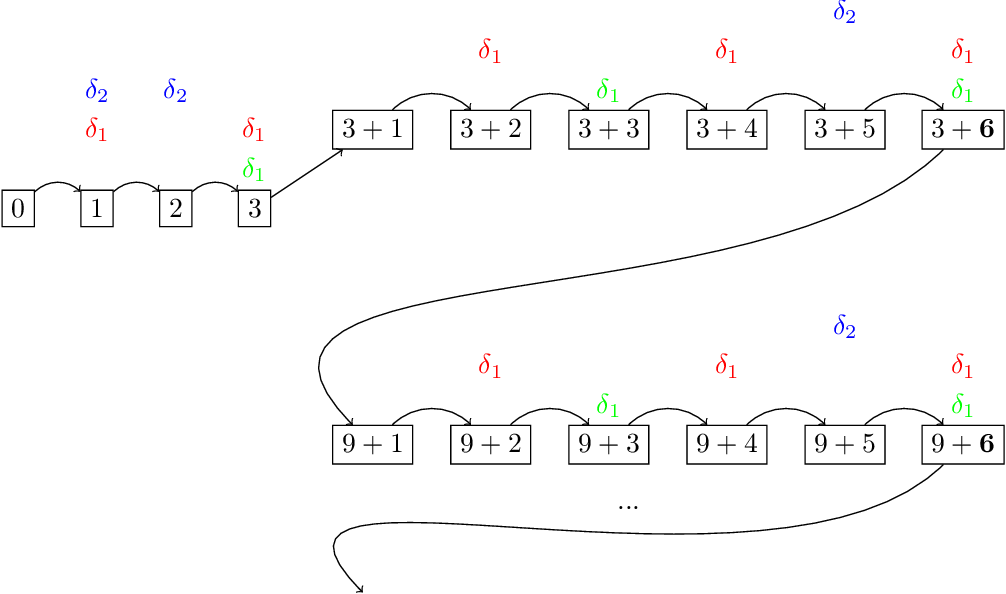Is this relevant?
2010
2010
• Theor. Comput. Sci.
• 2010
• Corpus ID: 17823643
We study decidability and complexity questions related to a continuous analogue of the Skolem-Pisot problem concerning the zeros… Expand
Is this relevant?
2006
2006
• J. Log. Comput.
• 2006
• Corpus ID: 1651434
A predicate logic of probability, close to the logics of probability of Halpern et al., is introduced. Our main result concerns… Expand
•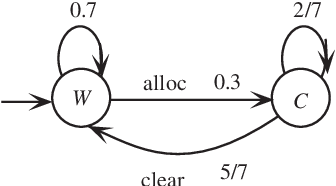•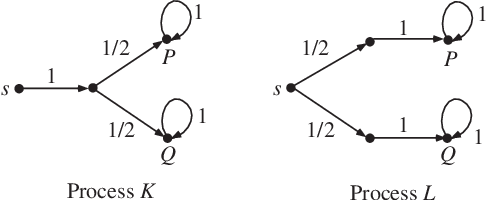•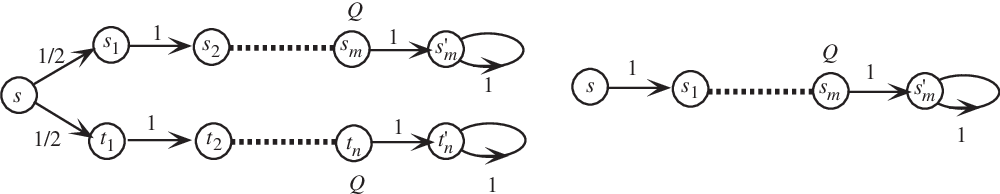Is this relevant?
1982
1982
The famous result of T. Skolem of 1933 assures the regularity of JA-sets of arbitrary integer valued matrices A. It prompts also… Expand
Is this relevant?
1981
1981
• Discret. Math.
• 1981
• Corpus ID: 11526664
A new relation between extended Skolem sequences (i.e., Skolem sequences with one zero element) and additive permutations is… Expand
Is this relevant?